Search
 Submission Procedure share: |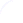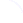available in:   PDF (101 kB) PS (32 kB)

get:
 Similar Docs BibTeX Write a comment
get:

DOI:   10.3217/jucs-002-05-0439

### ALGORITHMIC INFORMATION THEORY: OPEN PROBLEMS *1

Cristian Calude (Computer Science Department, The University of Auckland, Private Bag 92019, Auckland, New Zealand)
cristian@cs.auckland.ac.nz.

Abstract: This note collects some open problems in AIT which have been discussed during the Summer School ``Chaitin Complexity and Applications''. Each problem comes with the name(s) of the person(s) who suggested it, and more details about the listed open problems can be found directly by contacting the correspondent author: G. J. Chaitin (email: chaitin@watson.ibm.com) , F. Geurts (email: gf@info.ucl.ac.be) , H. Jürgensen (email: helmut@uwo.ca) , T. Odor (email: odor@math-inst.hu) , K. Svozil (email: svozil@tph.tuwien.ac.at) .
This list will be periodically up-to-dated in the home-page of the CDMTCS at url http://www.cs.auckland.ac.nz/CDMTCS/researchreports/ait.openproblems.ps.gz

1. Find questions in algebra, topology and geometry that are equivalent to determining bits of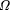. (G. J. Chaitin)

2. What is an interesting or natural mathematical question? (G. J. Chaitin)

3. How often is such a question independent of the usual axioms? (I suspect the answer is ``Quite often!'') (G. J. Chaitin)

4. Show that a classical open question in number theory, such as the Riemann hypothesis, is independent of the usual axioms. (I suspect that this is often the case, but that it cannot be proven.) (G. J. Chaitin)

5. The non-trivial zeros of Riemann's Zeta function are uniformly distributed, i.e. if we denote them by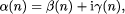then for every positive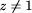, the sequence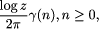is uniformly distributed modulo 1. Do non-trivial zeros of Riemann's Zeta function form a random sequence? (C. Calude)

6. When doing mathematics, should we take incompleteness seriously or is it a red herring? (I believe that we should take incompleteness very seriously indeed.) (G. J. Chaitin)

7. Is mathematics quasi-empirical? In other words, should mathematics be done more like physics is done? (I believe the answer to both questions is ``Yes.'') (G. J. Chaitin)

_________________

*1 C. Calude (ed.). The Finite, the Unbounded and the Infinite, Proceedings of the Summer School ``Chaitin Complexity and Applications'', Mangalia, Romania, 27 June -- 6 July, 1995.

Page 439

8. Further develop the theory of program-size complexity for infinite computations. Particularly, study the relations between program-size complexity and algorithmic probability. (G. J. Chaitin)

9. Chaitin complexity has been defined for finite strings. It would be interesting to extend it to infinite strings. Two ways can be envisaged:

-- (a) using non-terminating programs, looping forever, and generating infinite strings in an infinite amount of time;

-- (b) using computational models dealing with infinite strings in finite amounts of time (cellular automata, functions on reals, etc.). (F. Geurts) The first part has already received a small attention. The second one is probably another way to think about this complexity. (F. Geurts)

10. Is it possible to describe the ``deterministic chaos'' by means of Chaitin complexity? Discrete-time dynamical systems are called ``chaotic'' if they are sensitive to initial conditions. What is the relation between this definition (using topological transitivity and density of periodic points) and Chaitin complexity? (F. Geurts)

11. Study the transformations that preserve random strings and sequences. (C. Calude, H. Jürgensen)

12. Further develop algorithmic information theory for bi-infinite sequences and, more generally, for constructive cpo's. (C. Calude, H. Jürgensen) An idea would be to use bi-infinite cellular automata as computational models. (F. Geurts)

13. Every Chaitin random string is Kolmogorov random, but the converse is not true. Identify natural properties associated to ``randomness'' which are satisfied by Chaitin random strings, but not by Kolmogorov random strings. (C. Calude)

14. In a topological sense, ``many'' Kolmogorov random strings are not Chaitin random. Prove Solovay's Conjecture: There exists a constant L such that for all sufficiently large n, there are at least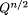strings of length n; s, such that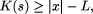and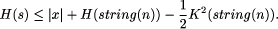(Here Q is the size of the underlying alphabet, H and K are, respectively, Chaitin and Kolmogorov-Chaitin complexities, cf. C. Calude, Information and Randomness, Springer, 1994.)

15. Axiomatize algorithmic information theory. (H. Jürgensen)

16. Constructive mathematics is essentially mathematics plus the assumption that ``existence = computability''. Constructions and objects in constructive mathematics have been studied from the point of view of dynamic complexities and it would be interesting to study them also from the point of view of program-size complexity. (C. Calude)

17. Derive incompleteness results for the determination of quantum algorithmic information. (K. Svozil)

18. Is there any model of computation in which the Invariance Theorem holds true also for the time complexity? (C. Calude)

19. Define and study the symmetry of random strings and sequences. Is the absence of symmetry a symptom of non-randomness? (C. Calude)

20. Study binary operations which preserve random strings/sequences. (T. Odor)

Page 440

21. Compute as many as possible bits of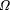. A possible way would be to run through all ``meaningful'', not all, programs. (C. Calude)

22. The degree of randomness of a string x depends upon the complexity of the string x compared with the complexities of all strings having the same length. Extend this definition of randomness ``with respect to an arbitrary finite set''. An appropriate solution should conform the following intuitive fact: if a finite set of strings M containing m elements, where m is very large, can be defined by a program of length negligible when compared with log m, then almost all elements of M should have maximal complexity (which is close to log m); these strings should be ``random'' with respect to M . (C. Calude)

Page 441